# Atomic Structure and Periodic TrendsPage 5

#### WATCH ALL SLIDES

Slide 38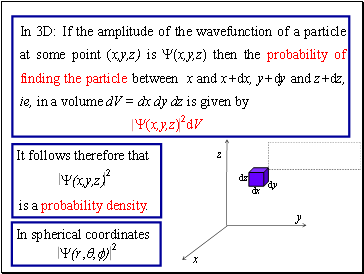In 3D: If the amplitude of the wavefunction of a particle at some point (x,y,z) is Y(x,y,z) then the probability of finding the particle between x and x+dx, y+dy and z+dz, ie, in a volume dV = dx dy dz is given by

Y(x,y,z) dV

dx

dy

dz

x

z

y

It follows therefore that

2

2

Y(x,y,z)

is a probability density.

In spherical coordinates

2

Y(r,q,f)

Slide 39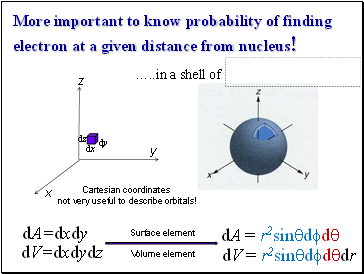More important to know probability of finding electron at a given distance from nucleus!

dx

dy

dz

x

z

y

dV=dxdydz

Cartesian coordinates

not very useful to describe orbitals!

… in a shell of

dA = r2sinqdfdq

dA=dxdy

dV = r2sinqdfdqdr

Surface element

Volume element

Slide 40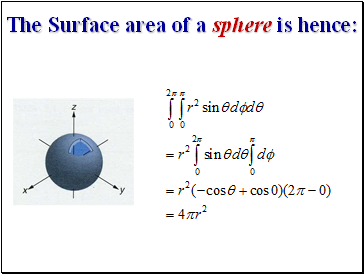## The Surface area of a sphere is hence:

Slide 41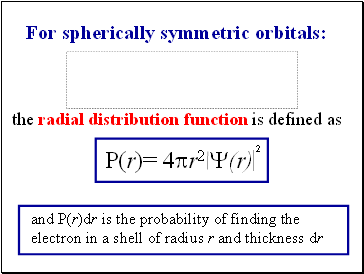For spherically symmetric orbitals:

the radial distribution function is defined as

2

P(r)= 4pr2 Y(r)

and P(r)dr is the probability of finding the electron in a shell of radius r and thickness dr

Slide 42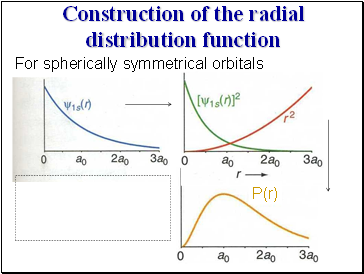## Construction of the radial distribution function

For spherically symmetrical orbitals

P(r)

Slide 43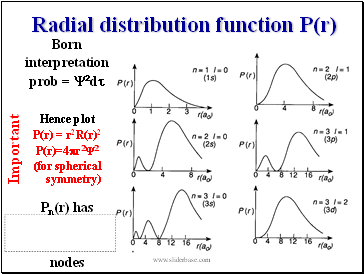Important

Born

interpretation

prob = Y2dt

Hence plot

P(r) = r2R(r)2

P(r)=4pr2Y2

(for spherical symmetry)

Pn(r) has

nodes

Slide 44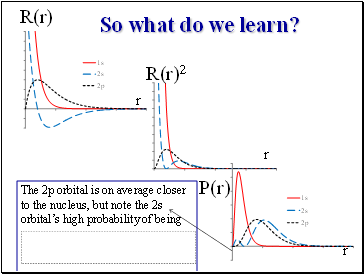So what do we learn?

R(r)

R(r)2

P(r)

r

r

r

The 2p orbital is on average closer to the nucleus, but note the 2s orbital’s high probability of being

Slide 45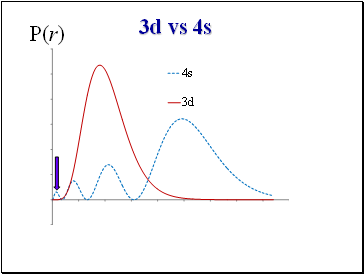3d vs 4s

P(r)

Slide 46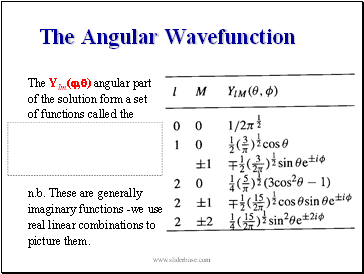## The Angular Wavefunction

The Ylm(,) angular part

of the solution form a set

of functions called the

n.b. These are generally

imaginary functions -we use

real linear combinations to

picture them.

Slide 47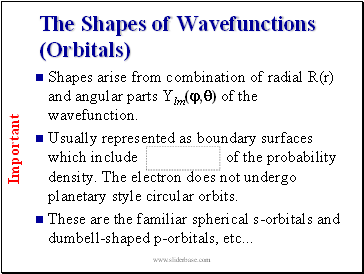## The Shapes of Wavefunctions (Orbitals)

Shapes arise from combination of radial R(r) and angular parts Ylm(,) of the wavefunction.

Usually represented as boundary surfaces which include of the probability density. The electron does not undergo planetary style circular orbits.

Go to page:
1  2  3  4  5  6  7  8  9  10  11  12  13  14  15
16  17